Next: Texture Compression Up: Parameter Estimation for Previous: Least Squares Estimate

## Maximum Likelihood Estimate of Parameters

We introduce the following approach as an improved method for estimating GMRF parameters of textured images. The method of maximum likelihood gives a better estimate of the texture parameters, since the asymptotic variance of the MLE is lower than that of the LSE. We also show a much faster algorithm for optimizing the joint probability density function which is an extension of the Newton-Raphson method and is also highly parallelizable.

Assuming a toroidal lattice representation for the image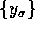and Gaussian structure for noise sequence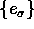, the joint probability density function is the following: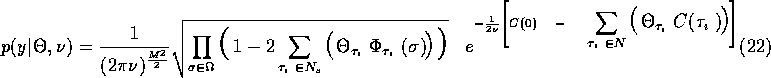In (22),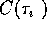is the sample correlation estimate at lag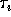. As described in  and , the log-likelihood function can be maximized: (Note that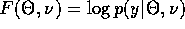).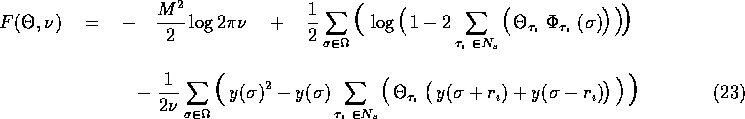For a square image,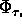is given as follows: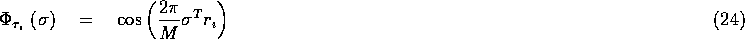This non-linear function F is maximized by using an extension of the Newton-Raphson method. This new method first generates a search direction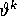by solving the system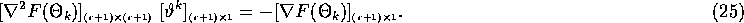Note that this method works well when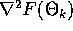is a symmetric, positive-definite Hessian matrix. We then maximize the step in the search direction, yielding an approximation to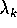which attains the local maximum of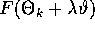and also satisfies the constraints that each of the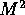values in the logarithm term for F is positive. Finally, an optimality test is performed. We set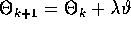, and if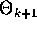is sufficiently close to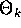, the procedure terminates. We give the first and second derivatives of F with respect toand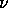in Appendix B.

For a rapid convergence of the Newton-Raphson method, it must be initialized with a good estimate of parameters close to the global maximum. We use the least squares estimate given in Subsection 4.1 as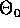, the starting value of the parameters.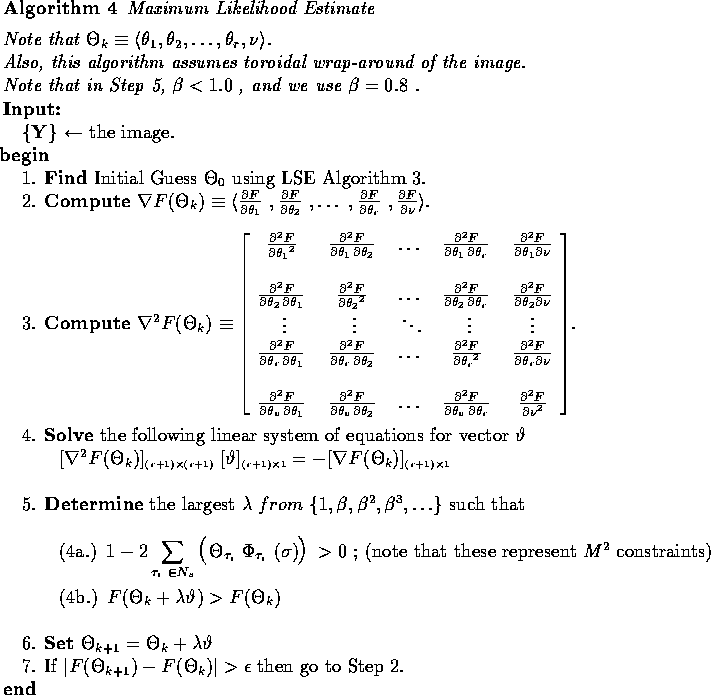The time complexities per iteration of the MLE algorithm are similar to that of the LSE algorithm analysis given in Subsection 4.1.

In Figures 6 - 8, we show the synthesis using least squares and maximum likelihood estimates for wool weave, wood grain, and tree bark, respectively, obtained from standard textures library. Tables 5, 6, and 7 show the respective parameters for both the LSE and MLE and give their log-likelihood function values. Each example shows that the maximum likelihood estimate improves the parameterization.

In addition, CM-5 timings for these estimates varying machine size, image size, and neighborhood models can be found in Tables 8, 9, 10, and 11, for a 4th order model on this selection of real world textured images, and in Tables 12, 13, 14, and 15, for a higher order model on the same set of images. Similarly, CM-2 timings for these estimates can be found in . Tables 8 - 15 are given in Appendix C.Next: Texture Compression Up: Parameter Estimation for Previous: Least Squares Estimate